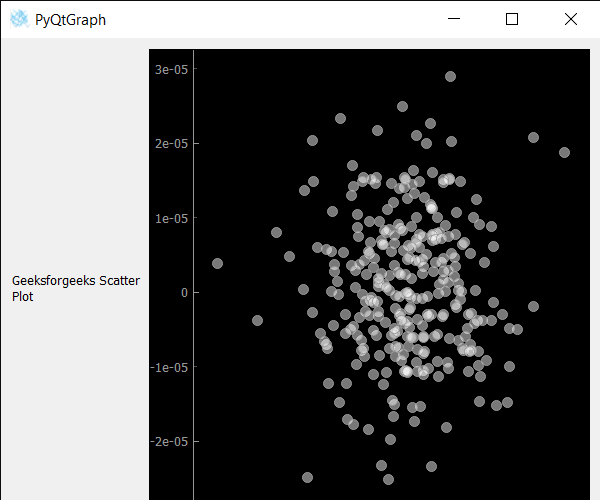# PyQtGraph – Scatter Plot Graph

• Last Updated : 18 Nov, 2021

In this article we will see how we can create a scatter plot graph using PyQtGraph module.  PyQtGraph is a graphics and user interface Python library for functionalities commonly required in designing and science applications. Its provides fast, interactive graphics for displaying data (plots, video, etc.). A scatter plot uses dots to represent values for two different numeric variables. It is a type of plot that uses Cartesian coordinates to display values for typically two variables for a set of data. The position of each dot on the horizontal and vertical axis indicates values for an individual data point. Scatter plots are used to observe relationships between variables.

We can create a plot window and create scatter plot graph on it with the help of commands given below

```# creating a pyqtgraph plot window
plt = pg.plot()

# creating a scatter plot graphof size = 10
scatter = pg.ScatterPlotItem(size=10)```

In order to create a scatter plot graph in pyqtgraph, following steps needs to be followed:

1. Import the pyqtgraph module
2. import other modules as well like numpy and pyqt5
3. Create a main window class
4. Create scatter plot item
5. Create random spots at random position using numpy
6. Add those spots to the scatter plot data
7. Create a grid layout
9. Set layout widget as the central widget

Example:

## Python3

 `# importing Qt widgets``from` `PyQt5.QtWidgets ``import` `*` `# importing system``import` `sys` `# importing numpy as np``import` `numpy as np` `# importing pyqtgraph as pg``import` `pyqtgraph as pg``from` `PyQt5.QtGui ``import` `*`  `class` `Window(QMainWindow):` `    ``def` `__init__(``self``):``        ``super``().__init__()` `        ``# setting title``        ``self``.setWindowTitle(``"PyQtGraph"``)` `        ``# setting geometry``        ``self``.setGeometry(``100``, ``100``, ``600``, ``500``)` `        ``# icon``        ``icon ``=` `QIcon(``"skin.png"``)` `        ``# setting icon to the window``        ``self``.setWindowIcon(icon)` `        ``# calling method``        ``self``.UiComponents()` `        ``# showing all the widgets``        ``self``.show()` `    ``# method for components``    ``def` `UiComponents(``self``):` `        ``# creating a widget object``        ``widget ``=` `QWidget()` `        ``# creating a label``        ``label ``=` `QLabel(``"Geeksforgeeks Scatter Plot"``)` `        ``# making label do word wrap``        ``label.setWordWrap(``True``)` `        ``# creating a plot window``        ``plot ``=` `pg.plot()` `        ``# number of points``        ``n ``=` `300` `        ``# creating a scatter plot item``        ``# of size = 10``        ``# using brush to enlarge the of white color with transparency is 50%``        ``scatter ``=` `pg.ScatterPlotItem(``            ``size``=``10``, brush``=``pg.mkBrush(``255``, ``255``, ``255``, ``120``))` `        ``# getting random position``        ``pos ``=` `np.random.normal(size``=``(``2``, n), scale``=``1e``-``5``)` `        ``# creating spots using the random position``        ``spots ``=` `[{``'pos'``: pos[:, i], ``'data'``: ``1``}``                 ``for` `i ``in` `range``(n)] ``+` `[{``'pos'``: [``0``, ``0``], ``'data'``: ``1``}]` `        ``# adding points to the scatter plot``        ``scatter.addPoints(spots)` `        ``# add item to plot window``        ``# adding scatter plot item to the plot window``        ``plot.addItem(scatter)` `        ``# Creating a grid layout``        ``layout ``=` `QGridLayout()` `        ``# minimum width value of the label``        ``label.setMinimumWidth(``130``)` `        ``# setting this layout to the widget``        ``widget.setLayout(layout)` `        ``# adding label in the layout``        ``layout.addWidget(label, ``1``, ``0``)` `        ``# plot window goes on right side, spanning 3 rows``        ``layout.addWidget(plot, ``0``, ``1``, ``3``, ``1``)` `        ``# setting this widget as central widget of the main window``        ``self``.setCentralWidget(widget)`  `# create pyqt5 app``App ``=` `QApplication(sys.argv)` `# create the instance of our Window``window ``=` `Window()` `# start the app``sys.exit(App.``exec``())`

Output :My Personal Notes arrow_drop_up